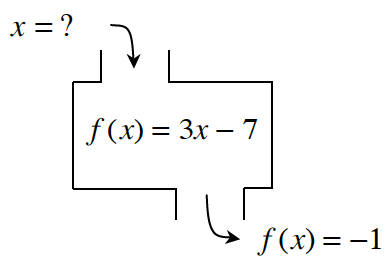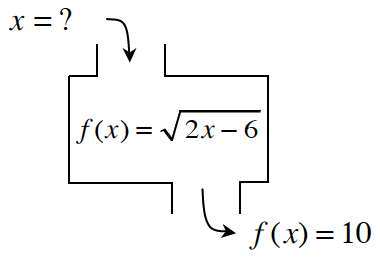### Home > CC4 > Chapter 1 > Lesson 1.3.2 > Problem1-82

1-82.

Determine the inputs for the following functions with the given outputs. If there is no possible input for the given output, explain why not. Homework Help ✎

1.Write out the equation.

$−1 = 3x − 7$

Solve for $x$.

$6 = 3x$

$2 = x$

1.Follow the steps in part (a).

$x = 53$